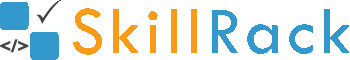ProgramID- 11190SkillRack
Largest among N Values

The program must accept N integers as the input. The program must print the largest integer among the N integers as the output.

Boundary Condition(s):
2 <= N <= 100
-1000 <= Each integer value <= 1000

Input Format:
The first line contains N.
The second line contains N integers separated by a space.

Output Format:
The first line contains the largest integer among the N integers.

Example Input/Output 1:
Input:
5
12 98 65 121 78

Output:
121

Explanation:
Here N = 5.
The largest integer among the 5 integers is 121.
Hence the output is 121

Example Input/Output 2:
Input:
4
-123 -9 -456 -3

Output:
-3

Max Execution Time Limit: 500 millisecs